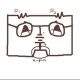Last few days I passed with Javascritp with its OOP concept. I found some beauties in Javascript programming language. So far everything we use in Javascript as function. But the object oriented concept is also available in the Javascript. Through Javascript is a class-less language but everything is an object in the Javascript. Basically, Javascript follows object-oriented, imperative, and functional programming styles as the same time.

First create an object that we can reuse in different purposes.

```var Rectangle = function (length, width) { this.length = length; this.width = width;   //Methods defined internally this.calcArea = function() { return this.length * this.width; };   this.getWidth = getWidth; //Methods defined externally: Anti pattern }   function getWidth(){ return this.width; }```

I create the object in constructor way. Now start using it..

```//Creating instance of Rectangle var myRectangle = new Rectangle(2, 4); console.log(myRectangle.calcArea()); console.log(myRectangle.getWidth());```

The outputs are:

```8 4```

You can create as much as new instance of Rectangle using this template. Now, method added as prototype. Let’s make it clear a bit. So far we have two methods one is calcArea() and another one is getWidth(). Both of the methods create when object is created but you want to add a new method to Rectangle (already Rectangle is constructed) that other objects of Rectangle can use that. Suppose we want to add a new method named: getLength. We will have to add getLength() to the prototype of the constructor function.

```Rectangle.prototype.getLength = function(){ return this.length; }```

Now call the new method..

`console.log(myRectangle.getLength());`

The output is:

`2`

Suppose a new object square has the same functionality as Rectangle has. We can again all methods to the square but it goes against the “DRY” principle of programming: Don’t Repeat Yourself. So, we will inherit square from Rectangle.

```var Square = function (length) { this.length = length; this.width = length; }   //For Inheriting all methods Square.prototype = new Rectangle();   //Creating instance of Square var mySquare = new Square(2); console.log(mySquare.calcArea());```

And, the output is:

`4`

Actually, Square using all the methods of Rectangle.

By the way, ECMAScript 5 introduced a new cool method: Object.create(). By calling the method, you can create a new object easily. The method accepts always the prototype of a object as its first argument.

So, you can also inherit in following way,

`Square.prototype = Object.create(Rectangle.prototype);`

Happy Learning *^_^*

## One thought on “Object Oriented In Javascript”

1.Md. Sadaf Noor says:

Thankyou vaia. Added in my learned list. 😀

This site uses Akismet to reduce spam. Learn how your comment data is processed.